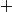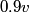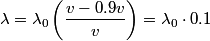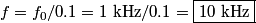## Solution to 1986 Problem 12

 The formula for the Doppler shift is:\begin{align*}\lambda = \lambda_0 \left(\frac{v \pm v_s}{v \pm v_o} \right)\end{align*}where the$+$ and$-$ signs are chosen depending on whether the source and observer are moving towards or away from each other. Here the observer (you) are not moving, and the source is moving towards you$0.9 v$, so\begin{align*}\lambda = \lambda_0 \left(\frac{v - 0.9 v}{v} \right) = \lambda_0 \cdot 0.1\end{align*}Inverting the equation, we find that,\begin{align*}f = f_0/0.1 = 1 \mbox{ kHz}/0.1 = \boxed{10 \mbox{ kHz}}\end{align*}Hence, answer (E) is the correct one.